# Solved 2016 Question Paper ICSE Class 10 Physics

## Section I (40 marks)

#### Question 1(a)

(i) Give an example of a non-contact force which is always of attractive nature.

(ii) How does the magnitude of this non-contact force on the two bodies depend on the distance of separation between them? 

(i) A non-contact force which is always of attractive nature is gravitational force.

(ii) Magnitude of non-contact forces on the two bodies is inversely proportional to the square of the distance of separation between them.

#### Question 1(b)

A boy weighing 40 kgf climbs up a stair of 30 steps each 20 cm high in 4 minute and a girl weighing 30 kgf does the same in 3 minutes. Compare :-

(i) The work done by them.

(ii) The power developed by them. 

(i) The work done by them = ?

While climbing both the girl and boy have to do work against the force of gravity.

Force of gravity on boy = F1 = m1g = 40 x 10 = 400

Force of gravity on girl = F2 = m2g = 30 x 10 = 300

Total height climbed up

h = number of steps x height of each step

= 30 x 20 = 600 cm = 6 m

Work done by the boy = W1 = F1 x h = 400 x 6 = 2400 J

Work done by the girl = W2 = F2 x h = 300 x 6 = 1800 J

Therefore, W1 : W2 = 2400 : 1800 = 4 : 3

(ii) The power developed by them = ?

$\dfrac{P_1}{P_2} = \dfrac{\dfrac{W_1}{t_1}}{{\dfrac{W_2}{t_2}}} \\[0.5em] = \dfrac{W_1}{W_2} \times \dfrac{t_2}{t_1} \\[0.5em] = \dfrac{4}{3} \times \dfrac{3}{4} \\[0.5em] = 1 : 1$

#### Question 1(c)

With reference to the terms Mechanical Advantage, Velocity Ratio and efficiency of a machine, name and define the term that will not change for a machine of a given design. 

The velocity ratio will not change.

Velocity ratio is defined as the ratio of the velocity of effort to the velocity of the load i.e.,

$\text {Velocity Ratio} = \dfrac{\text {Velocity of effort}}{\text {Velocity of load}} \\[0.5em]$

#### Question 1(d)

Calculate the mass of ice required to lower the temperature of 300 g of water at 40°C to water at 0°C. (Specific latent heat of ice = 336 J g-1, Specific heat capacity of water = 4.2 J g-1 °C-1) 

Given,

mass of water m = 300 g

initial temperature = 40°C

final temperature = 0°C

Therefore, fall in temperature = 40 - 0 = 40 °C

Heat lost by water = m x c x Δt

= 300 x 4.2 x 40 = 5.04 x 104 J

If m' g ice is added, heat gained by it to melt to 0 °C = m'L = m' x 336 J

By the principle of method of mixture,

heat lost by water = heat gained by ice

Therefore, 5.04 x 104 = m' x 336

m' = $\dfrac{5.04 \times 10^{4}}{336}$ = 150 g

#### Question 1(e)

What do you understand by the following statements :

(i) The heat capacity of the body is 60 J K-1.

(ii) The specific heat capacity of lead is 130 J kg-1 K-1 

(i) Heat energy required to raise the temperature of a body by 1 K is 60 J.

(ii) Heat energy required to raise the temperature of 1 Kg of lead by 1 K is 130 J.

#### Question 2(a)

State two factors upon which the heat absorbed by a body depends. 

The factors on which the quantity of heat absorbed by a body depend are :

1. The mass of the body,
2. The increase in temperature of the body.

#### Question 2(b)

A boy uses blue colour of light to find the refractive index of glass. He then repeats the experiment using red colour of light. Will the refractive index be the same or different in the two cases? Give a reason to support your answer. 

The speed of blue light in glass is less that of red light.

i.e. cb < cr

We know that,

$μ = \dfrac{\text{Speed of light in vacuum or air (c) }}{\text {Speed of light in glass (V)}} \\[0.5em]$

Hence, the refractive index of blue light is greater than that of red light.

i.e. μb > μr.

Hence, the refractive index of red and blue light in glass will be different.

#### Question 2(c)

Copy the diagram given below and complete the path of light ray till it emerges out of the prism. The critical angle of glass is 42°. In your diagram mark the angles wherever necessary.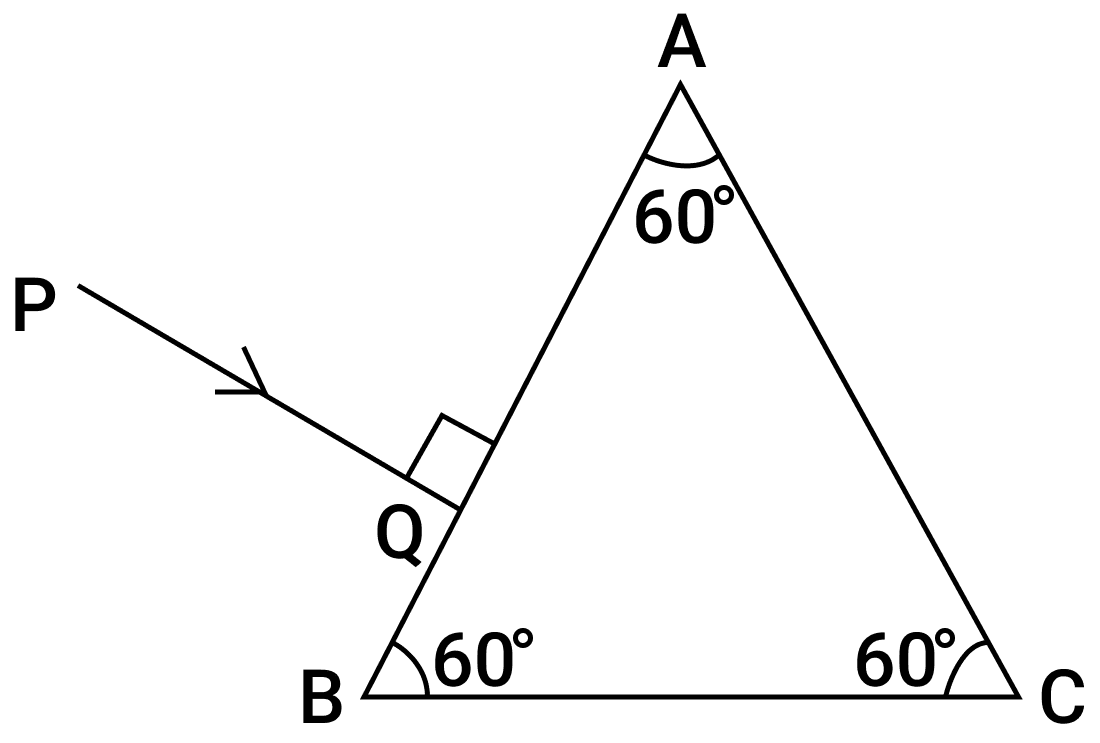Below diagram shows the complete path of light ray till it emerges out of the prism: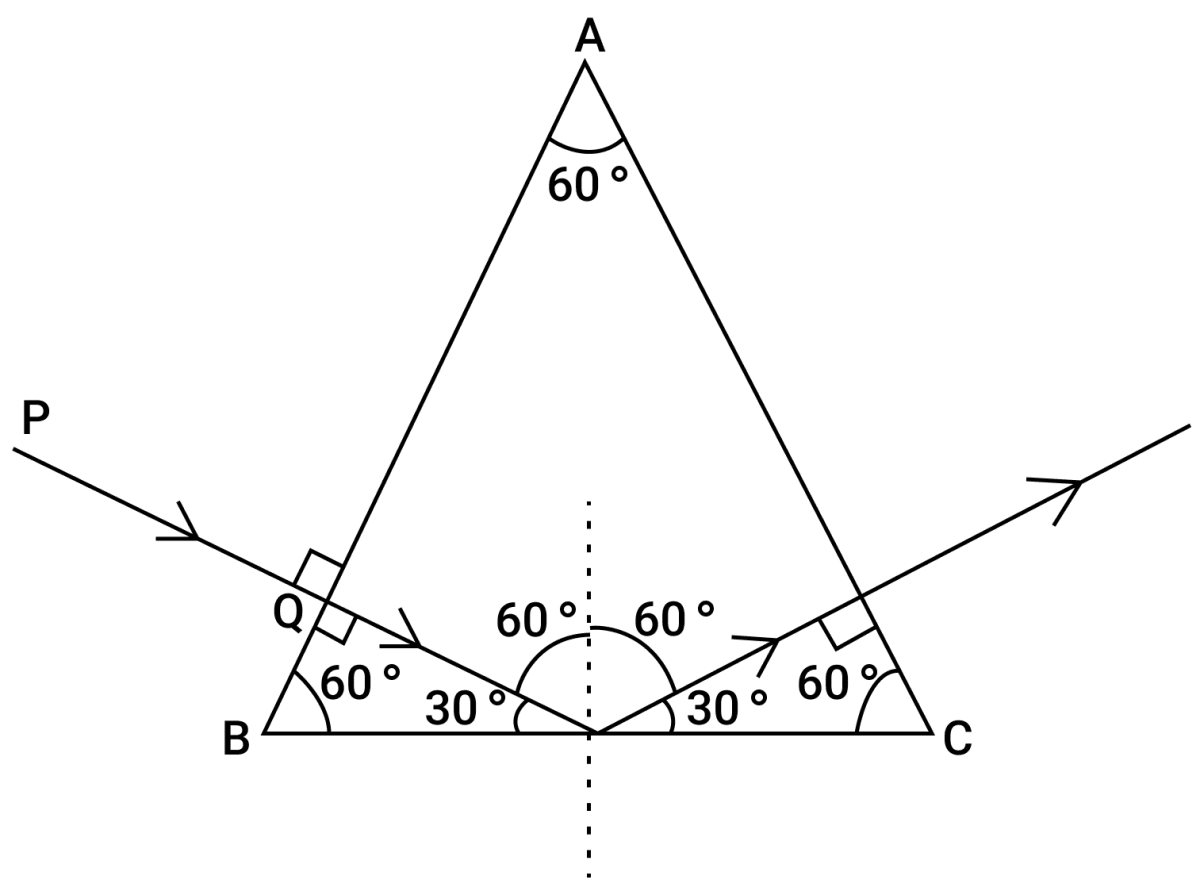#### Question 2(d)

State the dependence of angle of deviation :

(i) On the refractive index of the material of the prism.

(ii) On the wavelength of light. 

(i) For a given angle of incidence, the prism with a higher refractive index produces greater deviation than the prism which has a lower refractive index. Thus, the angle of deviation increases with an increase in the refractive index of the material of the prism.

(ii) Angle of deviation decreases with the increases in the wavelength of light.
Angle of Deviation (δ) ∝ $\dfrac{1}{\text{wavelength}}$

#### Question 2(e)

The ratio of amplitude of two waves is 3 : 4. What is the ratio of their :

(i) loudness?

(ii) frequencies? 

Given, a1 : a2 = 3 : 4

(i) Since, loudness ∝ (amplitude)2

Therefore,

$\dfrac{L_1}{L_2} = \Big(\dfrac{a_1}{a_2}\Big)^2 = \Big(\dfrac{3}{4}\Big)^2 = \dfrac{9}{16}$

(ii) The frequency of sound does not depend on it's amplitude, so the ratio of frequency f1 : f2 = 1 : 1

#### Question 3(a)

State two ways by which the frequency of transverse vibrations of a stretched string can be increased. 

The frequency of transverse vibrations of a stretched string can be increased :

1. Increasing the tension in the string.
2. Decreasing the length of the string.

#### Question 3(b)

What is meant by noise pollution? Name one source of sound causing noise pollution. 

Disturbances produced in the environment due to undesirable loud and harsh sound, of level above 120 dB is called noise pollution.

Examples of sources of noise pollution are — loudspeaker, siren, moving vehicles etc.

#### Question 3(c)

The V-I graph for a series combination and for a parallel combination of two resistors is shown in the figure below. Which of the two A or B, represents the parallel combination? Give a reason for your answer.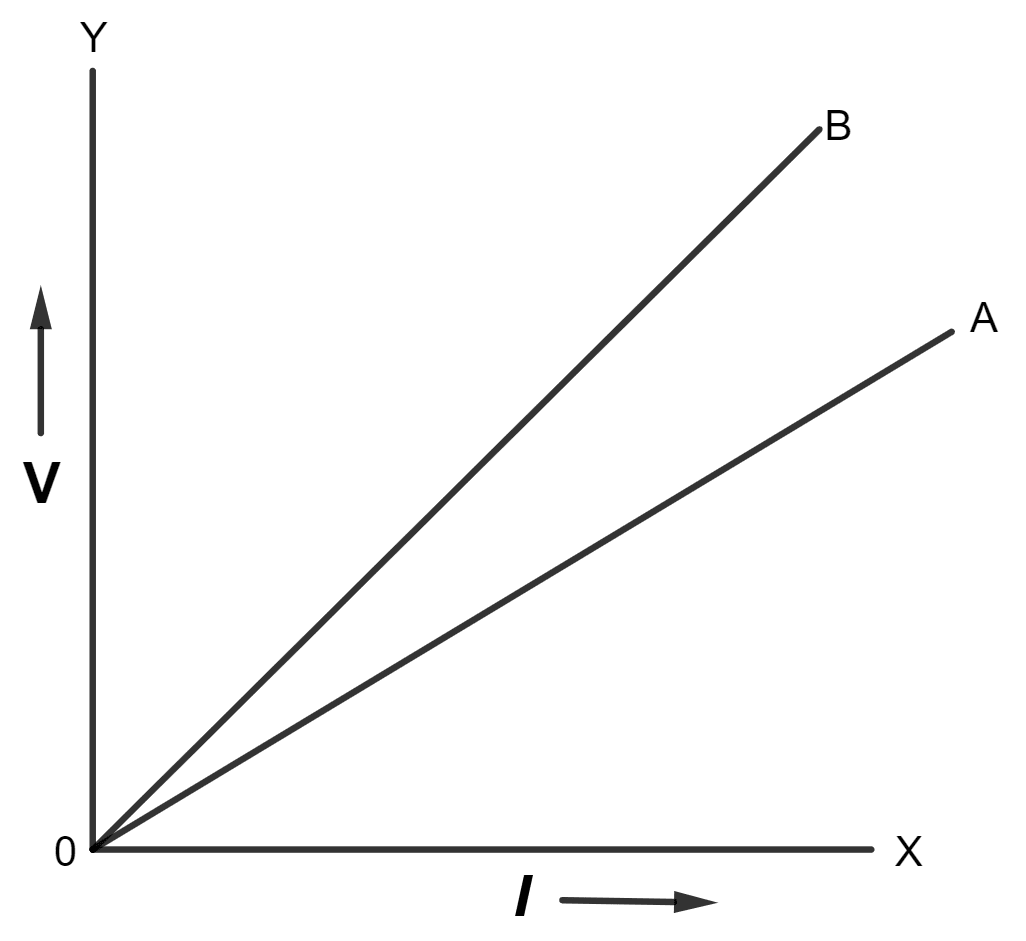The slope of V-I graph gives the resistance. Since the straight line A is less steeper than B, so the straight line A represents small resistance. In parallel combination, the equivalent resistance is less than in series combination so A represents the parallel combination.

#### Question 3(d)

A music system draws a current of 400 mA when connected to a 12 V battery.

(i) What is the resistance of the music system?

(ii) The music system is left playing for several hours and finally the battery voltage drops and the music system stops playing when the current drops to 320 mA. At what battery voltage does the music system stop playing? 

Given,

I = 400 mA = 400 x 10-3 = 0.4 A

V = 12 V

From Ohm's law,

V = IR

Substituting the values in the formula we get,

$12 = 0.4 \times R \\[0.5em] \Rightarrow R = \dfrac{12}{0.4} \\[0.5em] \Rightarrow R = 30 Ω$

Hence, the resistance of the music system = 30 Ω

(b) Given,

I = 320 mA = 320 x 10-3 A = 0.32 A

R = 30 Ω

From Ohm's law,

V = IR

Substituting the values in the formula we get,

V = 0.32 x 30 = 9.6V

Hence, the battery voltage when the music system stops playing = 9.6 V

#### Question 3(e)

Calculate the quantity of heat produced in a 20 Ω resistor carrying 2.5 A current in 5 minutes. 

Given, R = 20 Ω, I = 2.5 A, t = 5 min = 5 x 60 = 300 s

From relation H = I2Rt

Substituting the values we get,

H = (2.5)2 x 20 x 300 = 37500 J

#### Question 4(a)

State the characteristics required of a good thermion emitter. 

The characteristics required of a good thermion emitter are :

1. The work function of the substance should be low so that the electrons may be emitted from it even when it is not related to a high temperature.
2. The melting point of the substance should be high so that it may not melt when it is heated to the temperature required for thermionic emitter.

#### Question 4(b)

An element ZSA decays to 85R222 after emitting 2 α particles and 1 β particle. Find the atomic number and atomic mass of the element S. 

$_{85}\text{R}^{222}$ before the emission of β particle, would have atomic number 84, and before 2 α emissions, atomic number would have been 88. Hence, atomic number of X = 88.

$_{85}\text{R}^{222}$ before the emission of β particle, would have mass number 222, and before 2 α emissions, mass number would have been 230. Hence, mass number of X = 230.

#### Question 4(c)

A radioactive substance is oxidized. Will there be any change in the nature of it's radioactivity? Give a reason for your answer. 

There is no change in the nature of the radioactivity of the substance on being oxidized. It is so because radioactivity is a nuclear phenomenon so any physical change like oxidation does not affect the nature of radioactivity of the substance.

#### Question 4(d)

State the characteristics required in a material to be used as an effective fuse wire. 

The characteristics required in a material to be used as an effective fuse wire are :

1. The melting point should be low,
2. The specific resistance should be high.

#### Question 4(e)

Which coil of a step up transformer is made thicker and why? 

Primary Coil is made thicker than the secondary coil in a step up transformer. As current in primary coil is more than in the secondary coil so the wire in the primary coil is kept thicker.
The use of thicker wire in a coil reduces it's resistance and therefore, reduces the loss of energy as heat in that coil.

## Section II (40 Marks)

#### Question 5(a)

A stone of mass 'm' is rotated in a circular path with a uniform speed by tying a strong string with the help of your hand. Answer the following questions:

(i) Is the stone moving with a uniform or variable speed?

(ii) Is the stone moving with a uniform acceleration? In which direction does the acceleration act?

(iii) What kind of force acts on the hand and state it's direction? 

(i) The stone is moving with uniform speed.

(ii) Yes, the stone is moving with a uniform acceleration. The acceleration acts towards the centre.

(iii) Centrifugal force acts on the hand. It is directed radially outwards away from the centre.

#### Question 5(b)

From the diagram given below, answer the questions that follow: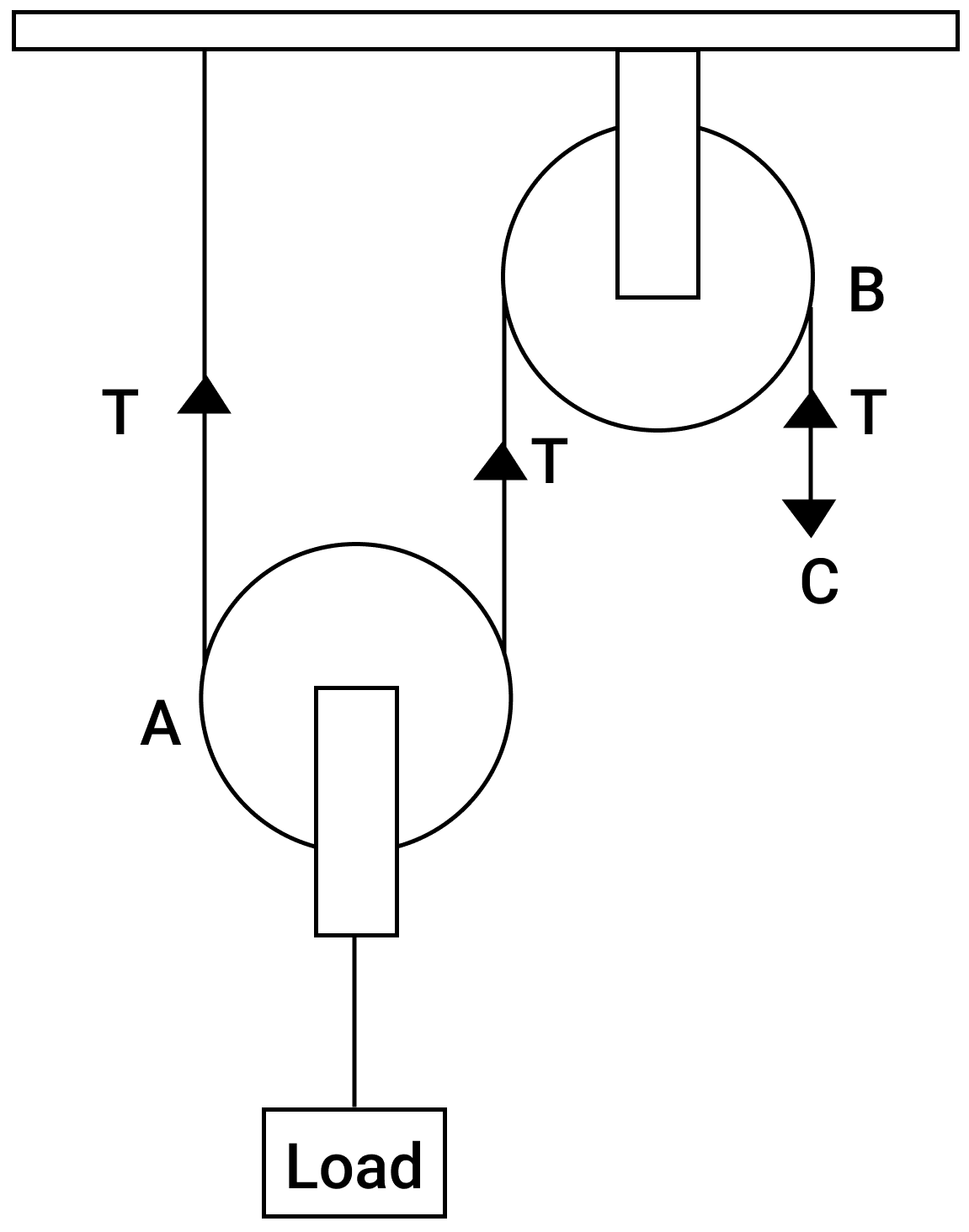(i) What kind of pulleys are A and B?

(ii) State the purpose of pulley B.

(iii) What effort has to be applied at C to just raise the load L = 20 kgf? 

(Neglect the weight of pulley A and friction).

(i) A is a movable pulley and B is a fixed pulley.

(ii) The purpose of pulley B is to change the direction of effort (i.e., in downward direction).

(iii) Given, Load = 20 kgf , M.A. = 2

From relation,

$\text{M.A.} = \dfrac{\text{L}}{\text{E}}$

Substituting the values in the formula above we get,

$2 = \dfrac{20}{\text{E}} \\[0.5em] \Rightarrow \text{E} = \dfrac{20}{2} \\[0.5em] \Rightarrow \text{E} = 10 \text{ kgf}$

#### Question 5(c)

(i) An effort is applied on the bigger wheel of a gear having 32 teeth. It is used to turn a wheel of 8 teeth. Where is it used?

(ii) A pulley system has three pulleys. A load of 120 N is overcome by applying an effort of 50 N. Calculate the Mechanical Advantage and Efficiency of this system. 

(i) In a gear system, the wheel on which effort is applied is called driving gear and other one is called driven gear. If number of teeth are more in driving gear than the driven gear, then we gain in speed.

(ii) Given, Load = 120 N and E = 50 N,

From relation,

$\text{M.A.} = \dfrac{\text{L}}{\text{E}}$

Substituting the values in the formula above we get,

$\text{M.A.} = \dfrac{120}{50} \\[0.5em] \Rightarrow \text{M.A.}= 2.4$

V.R. = 3

$\text{Efficiency (η)} = \dfrac{\text{M.A.}}{\text{V.R.}} \times 100%$

Substituting the values in the formula above we get,

$\text{η} = \dfrac{2.4}{3}\% \\[0.5em] \text{η} = 80\%$

#### Question 6(a)

(i) What is the principle of method of mixtures?

(ii) What is the other name given to it?

(iii) Name the law on which the principle is based. 

(i) According to principle of mixtures, heat lost by a hot body is always equal to the heat gained by a cold body provided no heat is lost to the surroundings.

(ii) The principle of method of mixtures is also called the principle of calorimetry.

(iii) It is based on the law of conservation of energy.

#### Question 6(b)

Some ice is heated at a constant rate, and it's temperature is recorded after every few seconds, till steam is formed at 100°C. Draw a temperature time graph to represent the change. Label the two phase changes in your graph. 

Temperature-time graph representing the change of ice to steam is shown below: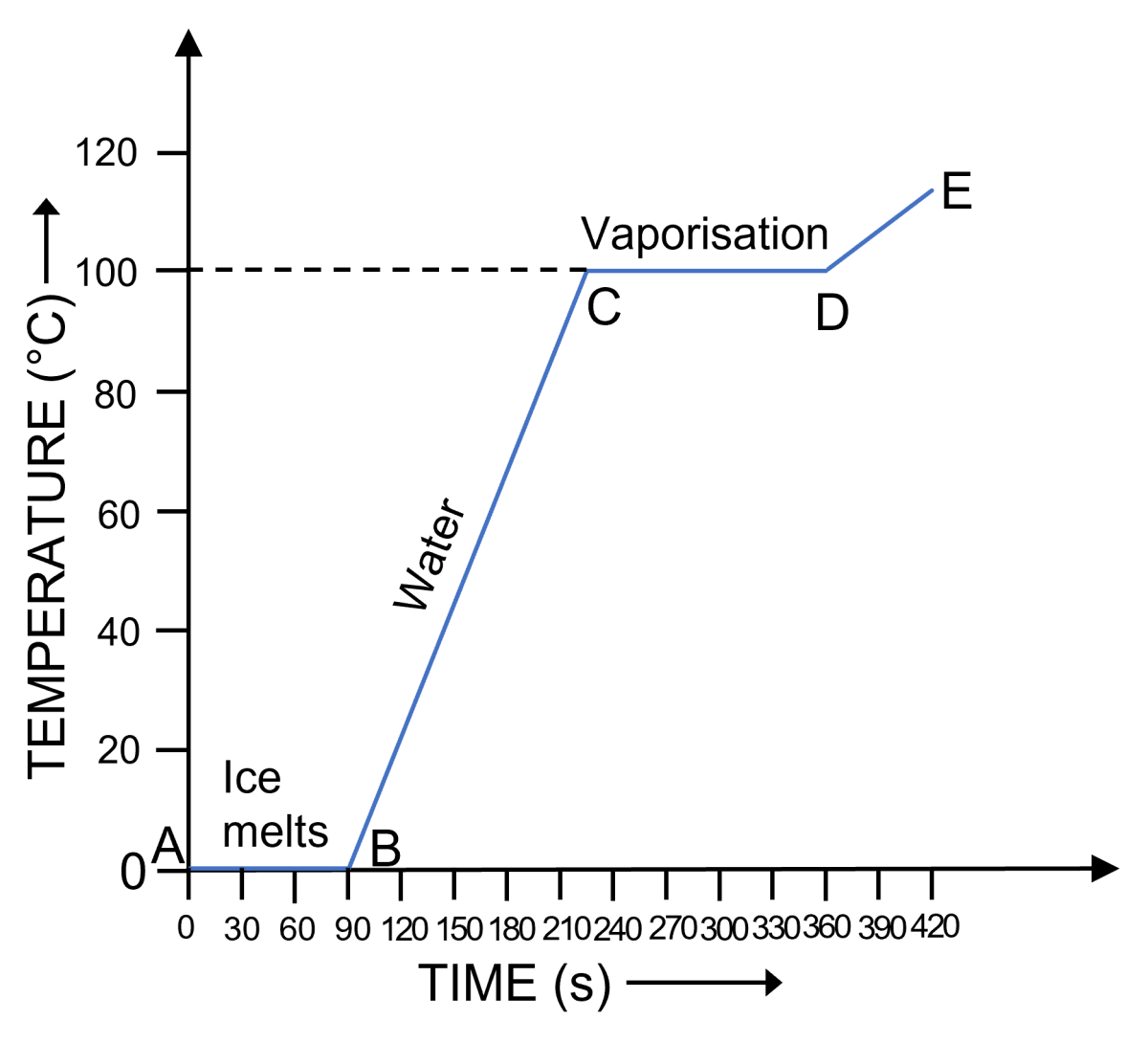#### Question 6(c)

A copper vessel of mass 100 g contains 150 g of water at 50 °C. How much ice is needed to cool it to 5 °C?

Given : Specific heat capacity of copper = 0.4 J g-1 ⁰C-1

Specific heat capacity of water = 4.2 J g-1 ⁰C-1

Specific latent heat of fusion of ice = 336 J g-1 

Heat energy imparted by vessel = 100 x 0.4 x (50 - 5) = 1800 J

Heat energy imparted by water = 150 x 4.2 x (50 - 5) = 28,350 J

Let m g of ice be used, then

Heat energy taken by ice to melt = m x 336 J

Heat energy taken by melted ice to raise it's temperature from 0 °C to 5 °C = m x 4.2 x (5 - 0) = 21m J

By law of conservation of energy

heat energy imparted by vessel and water = heat energy taken by ice and melted ice

1800 + 28,350 = 336m + 21m

357 m = 30,150

m = $\dfrac{30150}{357}$ = 84.45 g

#### Question 7(a)

(i) Write a relationship between angle of incidence and angle of refraction for a given pair of media.

(ii) When a ray of light enters from one medium to another having different optical densities it bends. Why does this phenomenon occur?

(iii) Write one condition where it does not bend when entering a medium of different optical density. 

(i) The ratio of the sine of angle of incidence 'i' to the sine of angle of refraction 'r' is constant for the pair of given media. This constant is called the refractive index.
1μ2 = $\dfrac{\text{sin i}}{\text{sin r}}$

(ii) It occurs due to change in speed of light as it enters from one medium to other medium having different optical density. If light slows down in the second medium, it bends towards the normal and if light speeds up, it bends away from the normal.

(iii) When the ray of light is incident normally on the surface of second medium, it does not bend and passes undeviated.

#### Question 7(b)

A lens produces a virtual image between the object and the lens.

(i) Name the lens.

(ii) Draw a ray diagram to show the formation of this image. 

(i) The lens is concave.

(ii) Below ray diagram shows the formation of this image: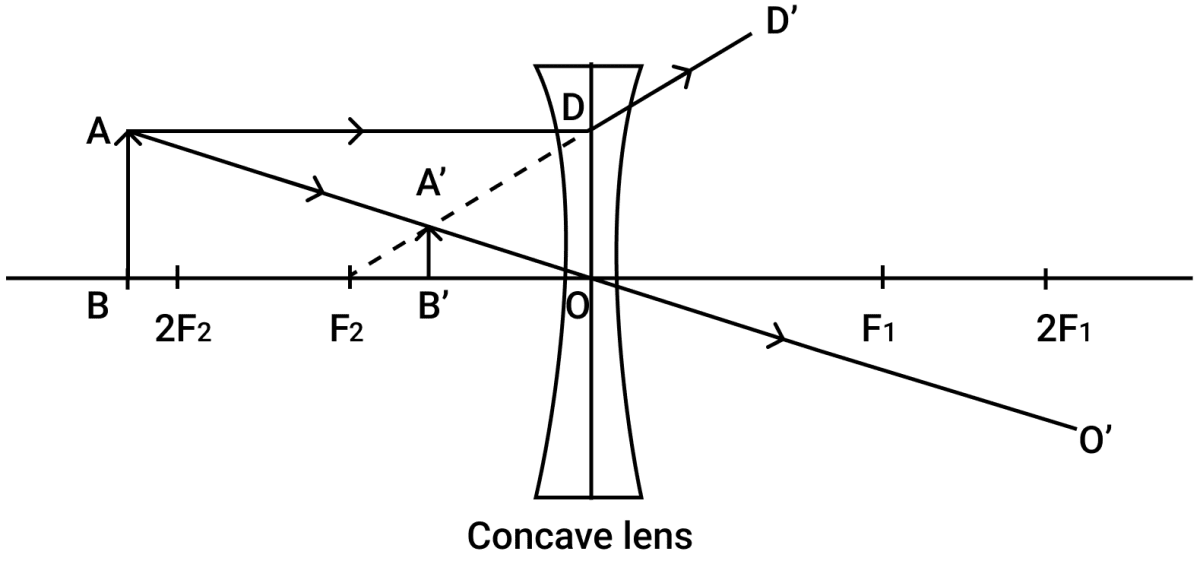#### Question 7(c)

What do you understand by the term 'Scattering of light'? Which colour of white light is scattered the least and why? 

Scattering is the process of absorption and then re-emission of light energy by the dust particles and air molecules present in the atmosphere.

Red colour of white light is scattered the least because wavelength of red light (λ = 8000 Å) is longest and intensity of scattered light (I) ∝ $\dfrac{1}{λ^4}$.

#### Question 8(a)

(i) Name the waves used for echo depth sounding.

(ii) Give one reason for their use for the above purpose.

(iii) Why are the waves mentioned by you not audible to us? 

(i) The waves used for echo depth sounding are ultrasonic waves.

(ii) They can travel undeviated through long distances.

(iii) Ultrasonic waves are not audible to us because they are of frequency above 20 kHz and the audible frequency range is 20 Hz to 20,000 Hz. Hence, we cannot hear ultrasonic waves.

#### Question 8(b)

(i) What is an echo?

(ii) State two conditions for an echo to take place. 

(i) The sound heard after reflection from a distinct obstacle (such as a cliff, a hillside etc.) after the original sound has ceased is called an echo.

(ii) Conditions for an echo to take place are :

1. The size of the reflector must be large enough as compared to the wavelength of the sound wave.
2. The intensity of sound should be such that the reflected sound reaching the ear is sufficiently loud to be audible.

#### Question 8(c)

(i) Name the phenomenon involved in tuning a radio set to a particular station.

(ii) Define the phenomenon named by you in part (i) above.

(iii) What do you understand by loudness of sound?

(iv) In which units is the loudness of sound measured? 

(i) Resonance.

(ii) Resonance is a special case of forced vibration. When the frequency of the externally applied periodic force on a body is equal to it's natural frequency, the body begins to vibrate with an increased amplitude. This phenomenon is known as resonance.

(iii) Loudness is the characteristic by virtue of which a loud sound can be distinguished from a faint one, both having the same pitch and quality.

(iv) The unit of loudness is phon. The level of sound is expressed in decibel (dB).

#### Question 9(a)

(i) Which particles are responsible for current in conductors?

(ii) To which wire of a cable in a power circuit should the metal case of a geyser be connected?

(iii) To which wire should the fuse be connected? 

(i) Electrons

(ii) Earth Wire

(iii) Live wire

#### Question 9(b)

(i) Name the transformer used in the power transmitting station of a power plant.

(ii) What type of current is transmitted from the power station?

(iii) At what voltage is this current available to our household? 

(i) Step-up transformer

(ii) Alternating current

(iii) 220 V

#### Question 9(c)

A battery of e.m.f. 12 V and internal resistance 2 Ω is connected with two resistors A and B of resistance 4 Ω and 6 Ω respectively joined in series.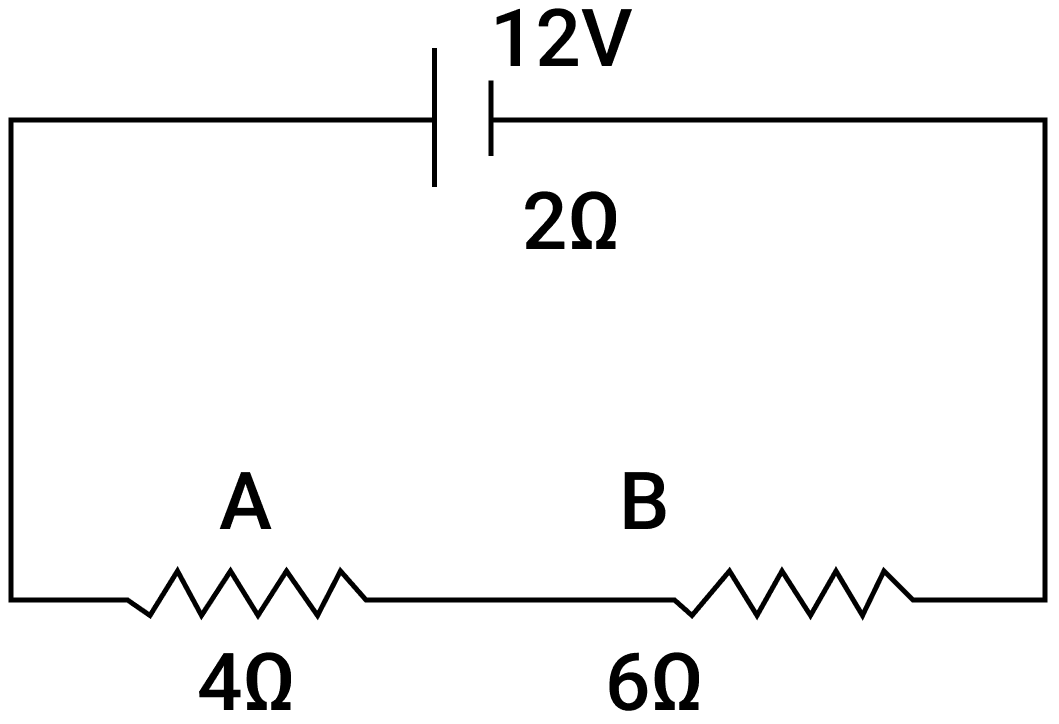Find:

(i) Current in the circuit.

(ii) The terminal voltage of the cell.

(iii) The potential difference across 6 Ω Resistor.

(iv) Electrical energy spent per minute in 4 Ω Resistor.

Given,

RA = 4 Ω

RB = 6 Ω

e.m.f. = 12 V

r = 2 Ω

(i) Two resistors ( 4 Ω and 6 Ω) are connected in series, so Rs = 4 + 6 = 10 Ω

From relation,

I = $\dfrac{𝐸}{𝑅 + 𝑟}$

Substituting the values we get,

$I = \dfrac{12}{10 + 2} \\[0.5em] I = \dfrac{12}{12} \\[0.5em] \Rightarrow I = 1 A$

(ii) Terminal voltage of cell V = ε - Ir

Substituting the values we get,

$V = 12 - (1 \times 2) \\[0.5em] V = 12 - 2 \\[0.5em] \Rightarrow V = 10 V$

(iii) P.d. across 6 Ω resistor = I R6Ω

Substituting the values we get,

V6Ω = 1 x 6 = 6 V

(iv) Electrical energy spent per minute (60 s) in 4 Ω Resistor = I2RAt = 1 x 6 = 6 V

#### Question 10(a)

Arrange α, β and γ rays in ascending order with respect to their

(i) Penetrating power.

(ii) Ionising power.

(iii) Biological effect. 

(I) Increasing order of penetrating power of α, β and γ rays is α<β<γ.

(ii) Increasing order of ionising power of α, β and γ rays is γ<β<α.

(iii) Increasing order of biological effect of α, β and γ rays is α<β<γ.

#### Question 10(b)

(i) In a cathode ray tube what is the function of anode?

(ii) State the energy conversion taking place in a cathode ray tube.

(iii) Write one use of cathode ray tube. 

(i) In a cathode ray tube, the anode accelerates the electrons and also focuses them in a fine energetic beam.

(ii) In a cathode ray tube, electric energy is converted into heat energy and then into light energy.

(iii) Cathode ray tube is used in oscilloscopes, which find their application in physics laboratories.

#### Question 10(c)

(i) Represent the change in the nucleus of a radioactive element when a β particle is emitted.

(ii) What is the name given to elements with same mass number and different atomic number?

(iii) Under which conditions does the nucleus of an atom tend to be radioactive? 

$\begin{matrix} _{Z}^{A}\text{P} & \longrightarrow & _{Z+1}^{\space \space \space \space \space A}\text{Q} & + & \space _{-1}^{\space \space \space 0}\text{e} \\ \footnotesize{\text{Parent}} & & \footnotesize{\text{Daughter}} & & \footnotesize{\text{Beta}} \\ \footnotesize{\text{nucleus}} & & \footnotesize{\text{nucleus}} & & \footnotesize{\text{particle}} \end{matrix}$